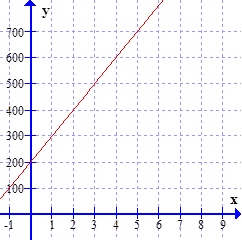### Sample Problem

A troop is recruiting soldiers and it accepts 50 new members every day. There are already 200 soldiers in the troop. If an equation were made to calculate the total number of soldiers in this troop, which of the following graphs would correctly depict the equation? (Check all that apply)

(A)(B)(C)(D)#### Solution

The equation would be C(x) = 50x + 200, where x is the number of days and 200 is the present number of soldiers. Thus, 50 is the slope and 200 is the y – intercept.